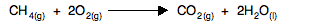Chemguide: Support for CIE A level Chemistry ``` ``` Learning outcome 23: Chemical energetics 23.4: Gibbs free energy change, ΔG ``` ``` Learning outcomes 23.4.1 and 23.4.2 These statements introduce the idea of Gibbs free energy change, and show how it can be calculated. Before you go on, you should find and read the statements in your copy of the syllabus. You won't be able to understand this topic unless you are already familiar with entropy changes from previous statements. ``` ``` Standard Gibbs free energy change, ΔG° Defining ΔG° For the purposes of these statements, I am just going to show how standard Gibbs free energy change is calculated. The importance of it will be dealt with in statements 23.4.3 and 24.4.4. ΔG° = ΔH° - TΔS° That's all you need to know. Learn it! ``` ``` Calculating ΔG° If you know (or can work out) the enthalpy change for the reaction, and you know (or can work out) the entropy change, and you know the temperature (in kelvin), then it would seem to be really easy to work out ΔG°. There is an easy mistake to be made though! You have to remember that the entropy change is calculated in energy units of joules, but ΔG° and ΔH° are both measured in kJ. You must remember to change the entropy change value into kJ before you start, otherwise you will get the calculation completely wrong. That might matter more than you think. In the final two statements in this section, you will find that the sign (positive or negative) of ΔG° matters. If you get that wrong in the calculation by not converting the entropy change value into kJ, it could mean that you couldn't complete the rest of a question properly. In statement 23.3.3, we calculated the entropy change for the reactionΔS° worked out as -242.2 J K-1mol-1. Before you do anything else, convert this to kJ by dividing by 1000. ΔS° = -0.2422 kJ K-1mol-1. This reaction is actually the combustion of methane, and so we can just take a value of this from a Data Book. But you should, of course, know how to calculate this from enthalpy changes of formation. ΔH° = -890.4 kJ mol-1. So if you had to calculate the Gibbs free energy change at, say, 298 K, you can just slot the numbers in: ΔG° = ΔH° - TΔS° ΔG° = -890.4 - 298(-0.2442) = -817.6 kJ mol-1 It is easy as long as you remember to convert the entropy change value into kJ. You will, of course, have to find some questions to practise on. ``` ``` Go to the Section 23 Menu . . . To return to the list of learning outcomes in Section 23 Go to the CIE Main Menu . . . To return to the list of all the CIE sections Go to Chemguide Main Menu . . . This will take you to the main part of Chemguide. © Jim Clark 2020